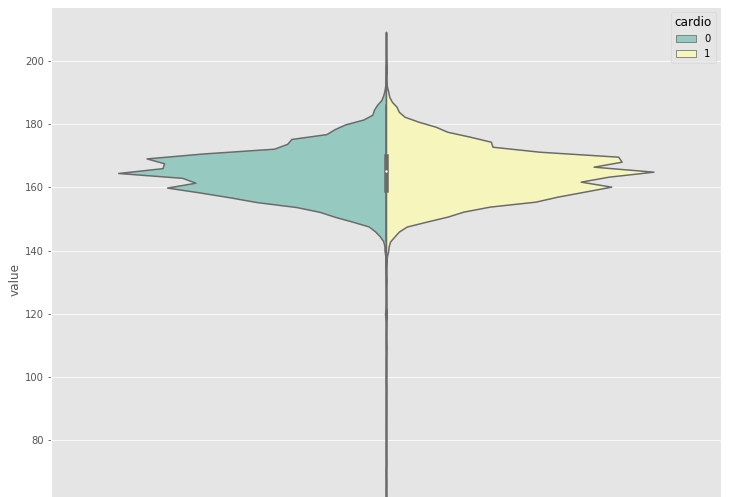Call Us @

(91) 96200 48623

## Blog: Predict Heart Attack using Machine Learning Tutorial- EDA, RF and GBMPredict Heart Attack using Machine Learning Tutorial- EDA, RF and GBM

"Heart disease is the leading cause of death for both men and women in the United States, accounting for about one million deaths each year." - source.

Preventive and predictive methods can help in managing the devastating effect of heart diseases. In this blog, we aim to show simple steps involved in building a predictive model using machine learning methods to predict a heart attack.

Building a system for heart attack prediction using machine learning, deep learning, and AI. Based on data available, the system/algorithm may be different.

For example, cardiovascular magnetic resonance (CMR) scans - image data along with patients' structured data (e.g. BP, Sugar, etc) can a powerful to predict accurately but the scans may not be available all the time. Also, it may be costly to ask non-high-risk patients.

Based structured information - factual (e.g. age, height, gender, weight, etc), medical examination results (e.g. BP, Glucose, etc), and behavioral/subjective given by patient (e.g. smoking, taking alcohol, level of physical activity, etc) could be a great stage one prediction system.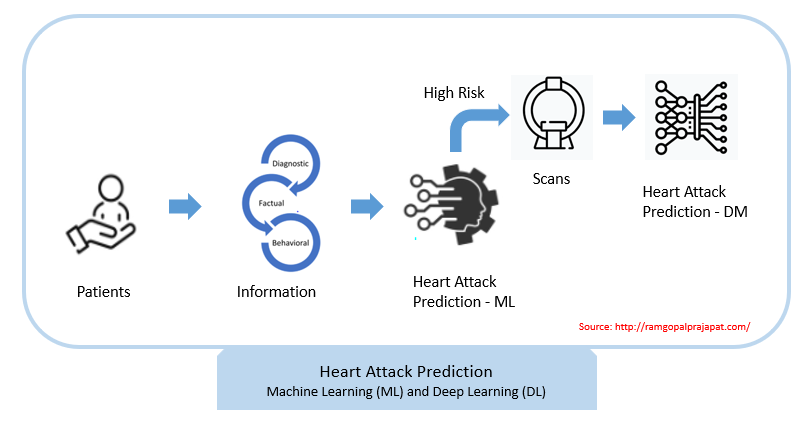## Data Overview

In this tutorial, we are going to use the heart attack prediction dataset available on Kaggle.

In this heart attack prediction dataset, structured information - factual (e.g. age, height, gender, weight, etc), medical examination results (e.g. BP, Glucose, etc), and behavioral/subjective given by patient (e.g. smoking, taking alcohol, level of physical activity, etc) - is available.

We will learn a process to build a machine learning-based heart attack prediction system. Over the process, we will learn

• Heart disease data set analysis - exploring the available features and their distributions

• Exploratory Data Analysis to answer questions like

• Is BP linked to increased heart disease risk?
• Is an increased glucose level causes a heart attack?
• The link between Cholesterol and Heart Attack
• Feature Engineering - creating new features based on existing ones. For example, difference & ratio of Systolic Blood Pressure and Diastolic Blood Pressure, BMI, and many more

• Heart Attack Prediction using Ranform Forest, Parameter Tuning and Performance Evaluation on Training & Testing datasets

• Heart Attack Prediction using XGBoost, Parameter Tuning and Performance Evaluation on Training & Testing datasets

```import pandas as pd cardio = pd.read_csv("cardio_train.csv", sep=";")```

`cardio.info()`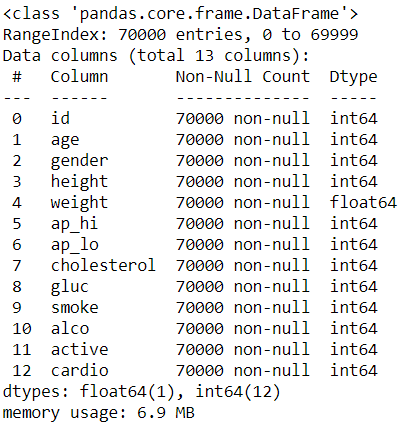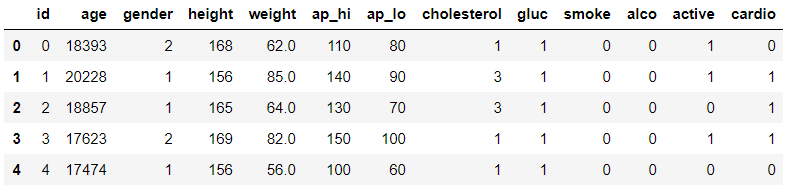Summary Statistics

cardio.describe()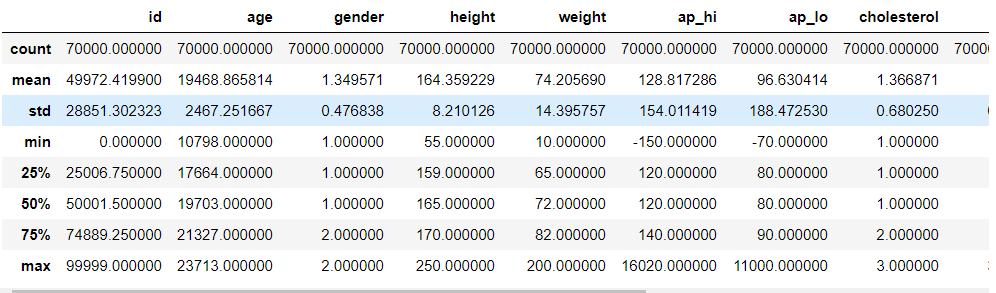Observations

• age: In days
• gender: Variable which takes value 1 and 2
• ap_hi: 75th percentile has 140 and the max value is 16020, so outliers or data issue. Similarly, on the lower side, the 25th percentile is 120 and the minimum is -150. So outliers and validate if negative values are acceptable
• ap_lo: Similar to ap_hi
• cholesterol: It is an ordinal variable with 3 levels.
• gluc: It is an ordinal variable with 3 levels.
• smoke: Ordinal variable with 28% rows are 1 and remaining are 0
• alco: Ordinal variable with 22% rows are 1 and remaining are 0
• active: Ordinal variable with 39% rows are 1 and remaining are 0

## Univariate Analysis

We have looked at summary statistics for each of the numeric variables. But detailed univariate analysis and visualization may be helpful to understand a bit more about the variable.

### Age

`# Plot Histogram`

`import matplotlib.pyplot as plt`

`fig, ax = plt.subplots()`

`ax.hist(cardio['age'],bins=15, cumulative=False)`

`ax.set_xlabel('Age (Days)')`

`ax.set_ylabel('Frequency')`

`plt.show()`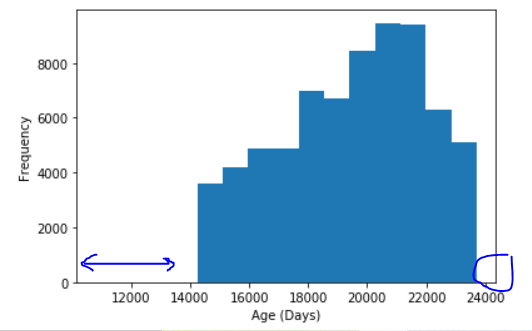`import matplotlib.pyplot as plt`

`df = pd.DataFrame(cardio, columns=['age'])`

`df.plot.box()`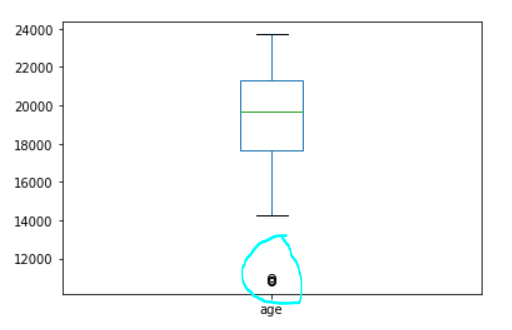The distribution looks a bit of confusing, though seems to have outliers. So may want to convert to years and see the top and bottom values.

`import numpy as np`

`cardio['age_years'] = round(cardio['age']/365,0)`

`# Plot Histogram`

`import matplotlib.pyplot as plt`

`fig, ax = plt.subplots()`

`ax.hist(cardio['age_years'],bins=15, cumulative=False)`

`ax.set_xlabel('Age (Years)')`

`ax.set_ylabel('Frequency')`

`plt.show()`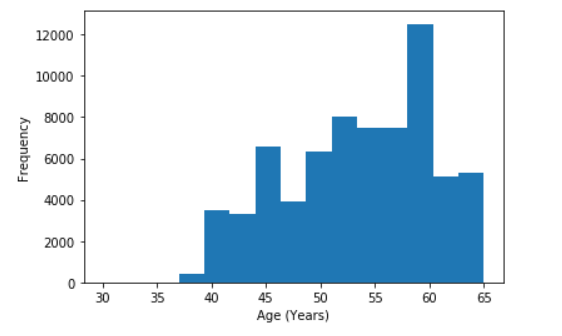`# Get lowest 10 values`

`cardio['age_years'].sort_values()[:10]`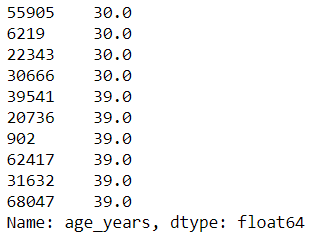Only a few patients have age 30; hence chart is depicted in this way. We can proceed for now without any action.

### Gender

`# Get counts for each gender`

`gender_summary=cardio.groupby('gender')['gender'].count()`

`gender_summary.index=['Women', 'Men']`

`# Get %`

`gender_pct = round(gender_summary*100/gender_summary.sum(),0)`

`# Bar Chart`

`import matplotlib.pyplot as plt`

`plt.style.use('ggplot')`

`plt.bar(gender_summary.index, gender_summary, color='green')`

`plt.xlabel("Gender")`

`plt.ylabel("Customer Counts")`

`plt.title("Patients by Gender")`

`plt.xticks(rotation=90) # change orientation of X axis tick label`

`# text on the top`

`for index, value in enumerate(gender_pct):`

`    plt.text(index,gender_summary[index]+value*10, str(int(value))+"%")`

`plt.show()`

`plt.close()`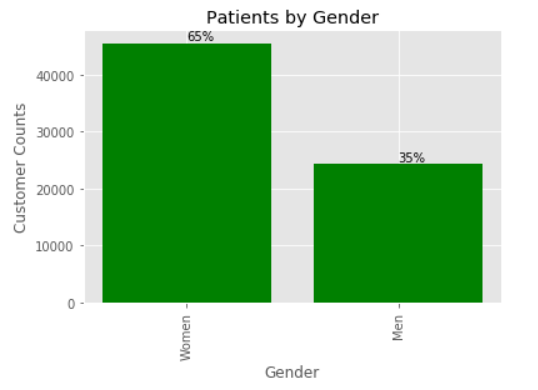Observation: The sample has significantly higher representations of "Female" patients.

### Height

Height of Patients in cm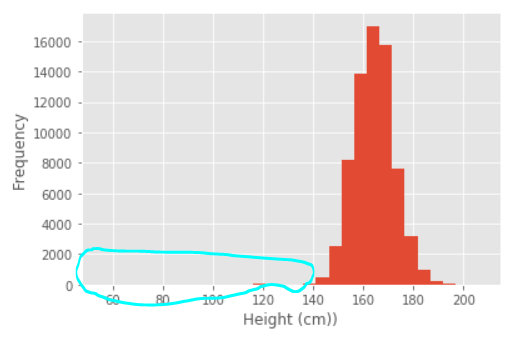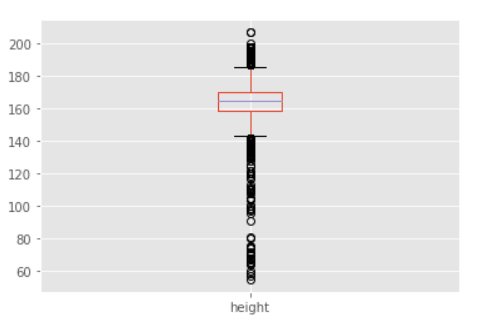Observations:

• On the higher side of height, one patient has an abnormally higher height of -250 cm. We can cap this to 207
• On the lower side of height, there are a few patients with low heights. We can create a feature which captures this group of patients

`import numpy as np`

`cardio['height'] = np.where(cardio['height']>207,207,cardio['height'])`

`cardio['height'].sort_values()[-10:]`

### Weight

Weight of Patients in Kg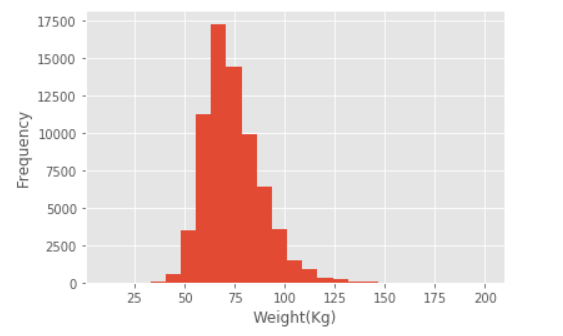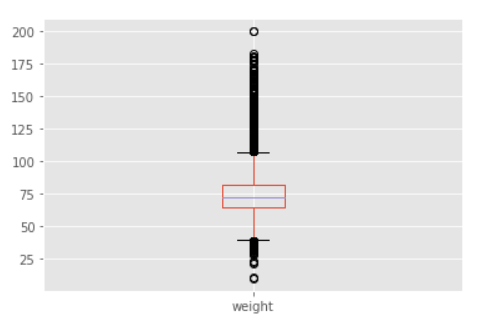Observation: Nice close to the normal distribution of the weights. There are a few values that are a bit away from the median but not a major issue.

### Systolic Blood Pressure

Systolic blood pressure (the first number) – indicates how much pressure your blood is exerting against your artery walls when the heartbeats.

"Systolic blood pressure (the first number) as a major risk factor for cardiovascular disease for people over 50"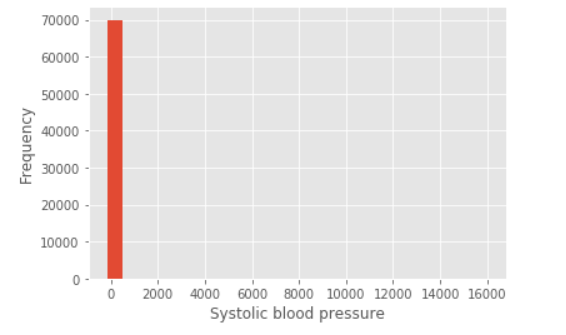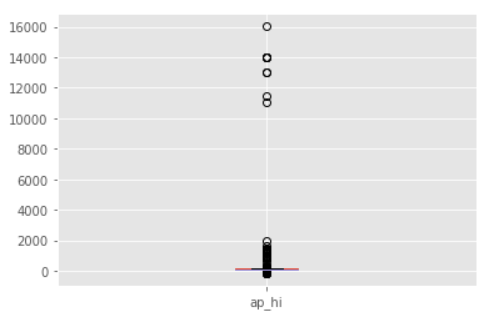Observations:

• Major outliers and not sure if these are even possible values as the normal value is only 120.
• Probably, two scenarios, issue with the measurement or real high valid values. So creating a categorical variable to capture this behavior may be a good option.
• Cap the values to 201 - if higher value then replaces with 201

`# Create multiple group using lamda function`

`def ap (values):`

`    if values<=120:`

`        return 1`

`    elif 120<values<=200:`

`        return 2`

`    else:`

`        return 3`

`cardio['ap_hi_cat']=cardio.ap_hi.apply(lambda x: ap(x) )`

`cardio['ap_hi_cat'].value_counts()`

`import numpy as np`

`cardio['ap_hi'] = np.where(cardio['ap_hi']>200,201,cardio['ap_hi'])`

`# See distribution now`

`# Plot Histogram`

`import matplotlib.pyplot as plt`

`fig, ax = plt.subplots()`

`ax.hist(cardio['ap_hi'],bins=25, cumulative=False)`

`ax.set_xlabel('Systolic blood pressure')`

`ax.set_ylabel('Frequency')`

`plt.show()`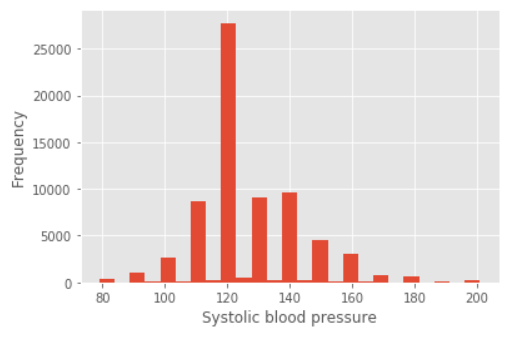Observation Outlier toward the lower side now. So, we may need to do the treatment here as well. Only a few observations, so we may not create an additional variable to capture this behavior

`import numpy as np`

`cardio['ap_hi'] = np.where(cardio['ap_hi']<80,79,cardio['ap_hi']).`

### Diastolic Blood Pressure¶

Diastolic blood pressure (the second number) – indicates how much pressure your blood is exerting against your artery walls while the heart is resting between beats.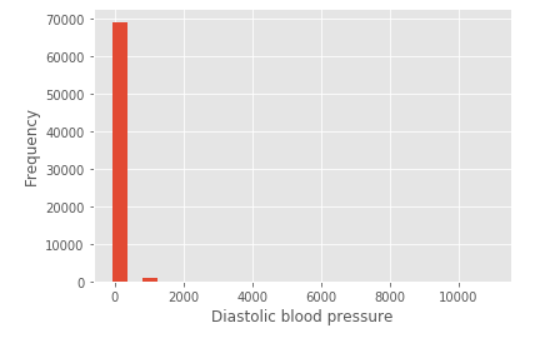`# Create multiple group using lamda function`

`def aplow (values):`

`    if values<=50:`

`        return 1`

`    elif 50<values<=120:`

`        return 2`

`    else:`

`        return 3`

`cardio['ap_lo_cat']=cardio.ap_lo.apply(lambda x: aplow(x) )`

`# Capping`

`def capping(series, lowMax, highMin):`

`    if series <lowMax:`

`        return lowMax`

`    elif series>highMin:`

`        return highMin`

`    else:`

`        return series`

`cardio['ap_lo'] = cardio.ap_lo.apply(lambda x: capping(x,50,120) )`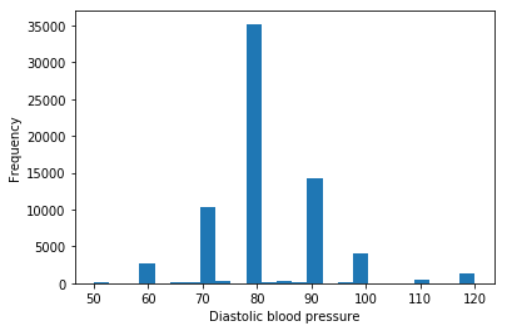Observation

• Mostly values are like a multiplier of tens. But a few counts seem to be between. So creating a new categorical variable to capture this.

`import numpy as np`

`cardio['ap_lo_mod_10'] = np.where(cardio['ap_lo']%10==0,1,0)`

`cardio['ap_lo_mod_10'].value_counts()`

Cholesterol

Cholesterol is a risk factor for heart disease, but recent research suggests the connection may be more complex. In this data sample, the column takes values 1, 2, and 3.

• 1: normal
• 2: above normal
• 3: well above normal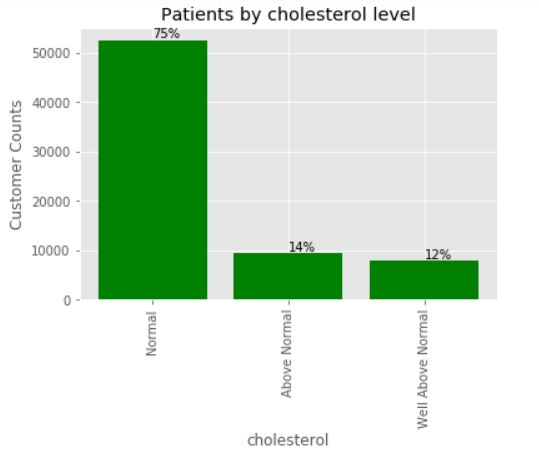26% of the patients have a higher cholesterol level. Based on the data, we will see if there are a higher contribution heart disease for this group.

### Glucose

Over time, high blood glucose from diabetes can damage your blood vessels and the nerves that control your heart and blood vessels. The longer you have diabetes, the higher the chances that you will develop heart disease. Source

For this sample, it is a categorical variable and 3 levels are 1: normal, 2: above normal, 3: well above normal.

Though, a time history of high glucose is not available.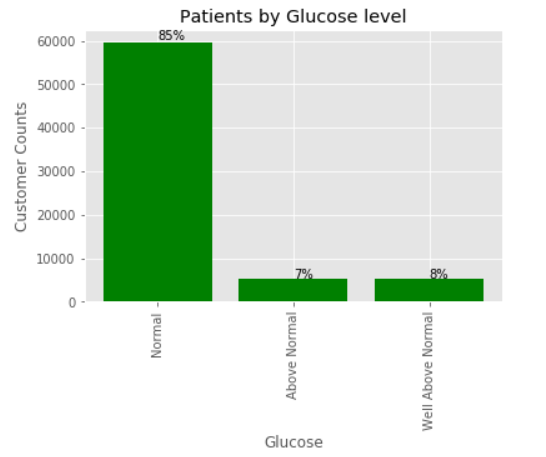15% of the patients have a higher glucose level.

### Smoking

Whether a patient smokes or not.

# Create summary table

smoke_summary=cardio.groupby('smoke')['smoke'].count()

# Pie Chart

import matplotlib.pyplot as plt

label = 'Non Smokers','Smokers'

plt.pie(smoke_summary,labels=label,autopct='%1.1f%%')

plt.title('Smoking')

plt.axis('equal')

plt.show()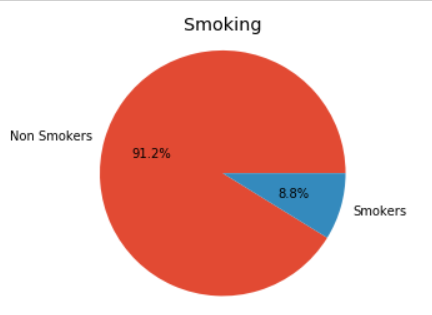### Alcohol Intake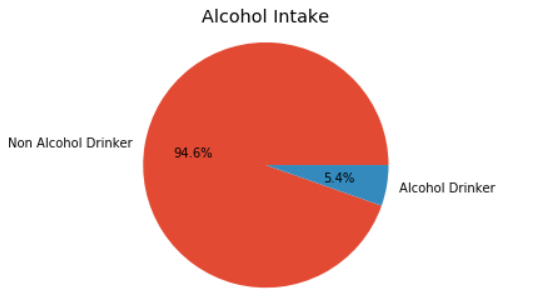### Physical activity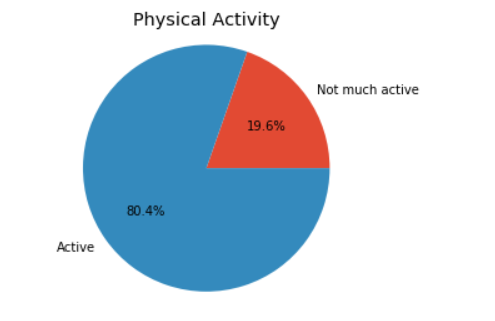###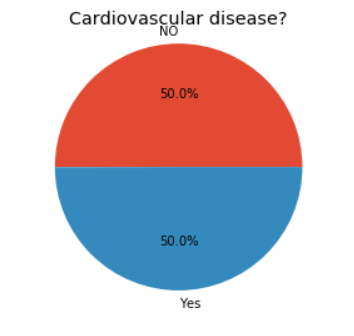Feature Engineering: BMI

The data sample has the patients' weight and height. One of the first features, we can create is BMI. One hypothesis is that higher BMI is linked to a higher risk of heart disease.

BMI = weight (kg) / [height (m)]2

BMI Ranges

• Underweight = <18.5
• Normal weight = 18.5–24.9
• Overweight = 25–29.9
• Obesity = BMI of 30 or greater

`# BMI Cal`

`cardio['bmi'] = np.round(cardio['weight']/((cardio['height']/100)*(cardio['height']/100)),0)`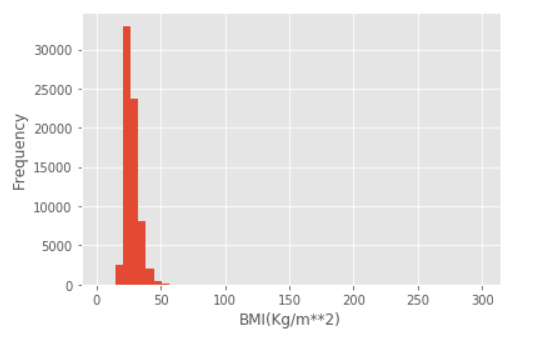`# Outlier Treatment`

`import numpy as np`

`cardio['bmi'] = np.where(cardio['bmi']>50,50,cardio['bmi'])`

`# Create multiple group using lamda function`

`def bmicat(values):`

`    if values <=18.5:`

`        return 1`

`    elif 18.5<values<=24.9:`

`        return 2`

`    elif 24.9<values<=29.9:`

`        return 3`

`    else:`

`        return 4`

`   `

`# Create categorical variable`

`cardio['bmi_cat'] = cardio.bmi.apply(lambda x: bmicat(x) )`

`# Get counts for each gender`

`bmi_cat_summary=cardio.groupby('bmi_cat')['bmi_cat'].count()`

`bmi_cat_summary.index=['Underweight', 'Normal Weight', "Overweight","Obesity"]`

`# Get %`

`bmi_pct = round(bmi_cat_summary*100/bmi_cat_summary.sum(),0)`

`# Bar Chart`

`import matplotlib.pyplot as plt`

`plt.style.use('ggplot')`

`plt.bar(bmi_cat_summary.index, bmi_cat_summary, color='green')`

`plt.xlabel("BMI")`

`plt.ylabel("Customer Counts")`

`plt.title("Patients by BMI level")`

`plt.xticks(rotation=90) # change orientation of X axis tick label`

`# text on the top`

`for index, value in enumerate(bmi_pct):`

`    plt.text(index,bmi_cat_summary[index]+value*10, str(int(value))+"%")`

`plt.show()`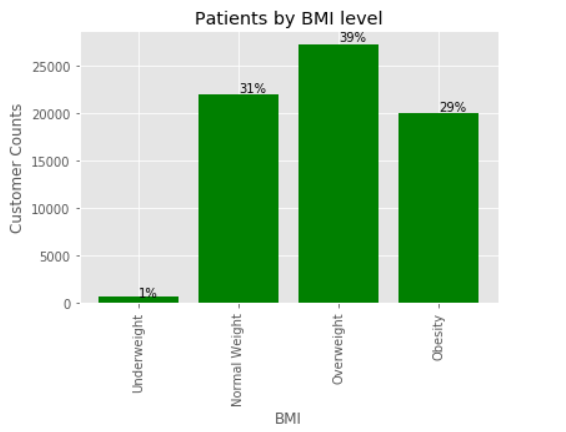### Feature Engineering: Ratio and Difference of Pressures

The ration of diastolic to systolic blood pressures can be a useful feature to consider. Similarly, we can find the difference and assess if that is linked to the disease.

`cardio['s_d_ratio'] = np.round(cardio['ap_hi']/cardio['ap_lo'],2)`

`cardio['s_d_diff'] = np.round(cardio['ap_hi']-cardio['ap_lo'],2)`

## Bivariate Analysis

Now, the relationship between the target/label variable and each of the features is explored. We can find the scale of measurement to find the right tool to be used.

### Bivariate: BMI

`import warnings`

`warnings.filterwarnings("ignore")`

`import seaborn as sns`

`cardio_melt = pd.melt(frame=cardio, value_vars=['bmi'], id_vars=['cardio'])`

`plt.figure(figsize=(12, 10))`

`ax = sns.violinplot(`

`    x='variable',`

`    y='value',`

`    hue='cardio',`

`    split=True,`

`    data=cardio_melt,`

`    scale='count',`

`    scale_hue=False,`

`    palette="Set3");`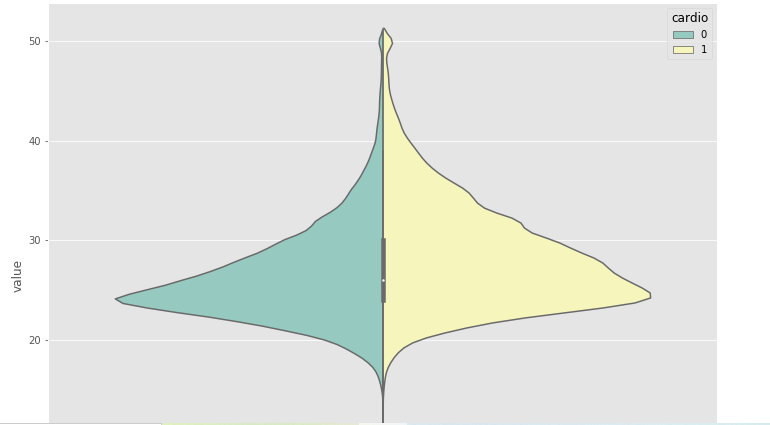### Bivariate: Age (Years)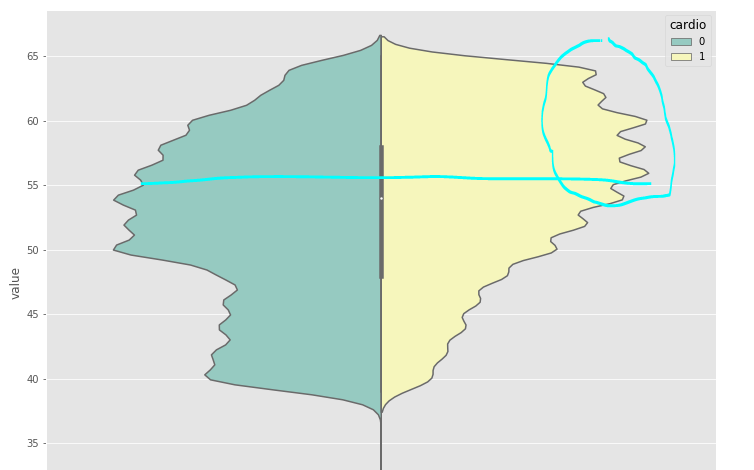Observation

• Some differences in the distribution. Cardio=1 is skewed toward higher age values. This is as per expectations.
• After age 55, there is a remarkable difference
• Age groups can be created and potentially use those as independent variables.

### Ratio of Pressures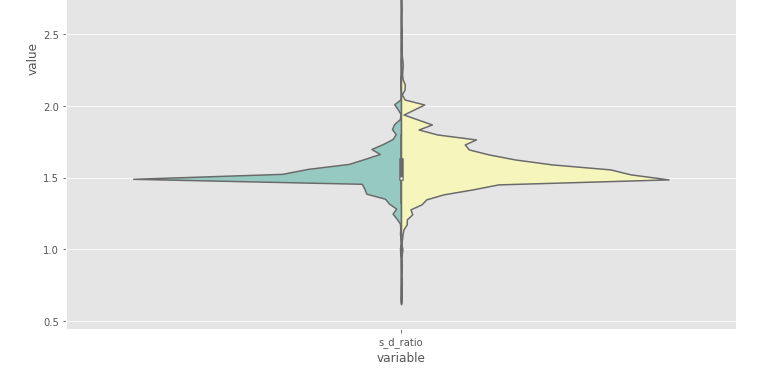### Difference of Pressures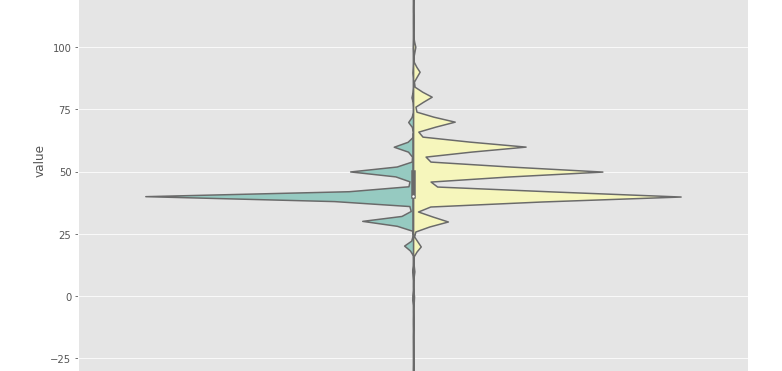Observation

The difference in pressure has some association to the disease based on the above distributions.

### Diastolic Blood Pressure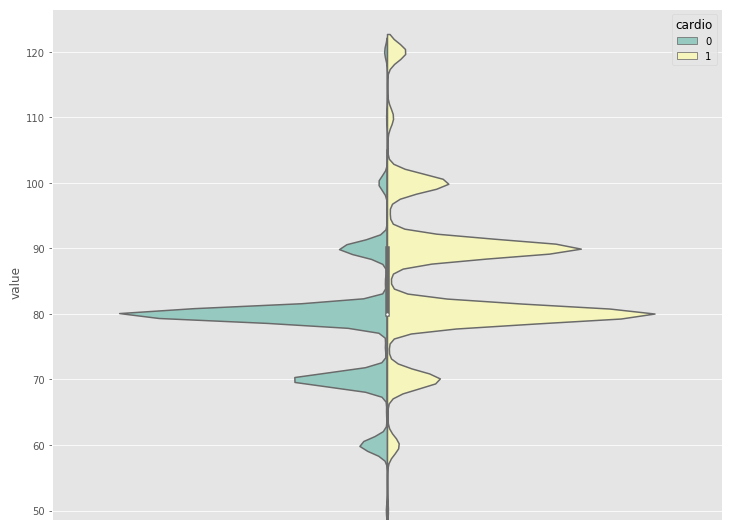Diastolic Blood Pressure seems to be linked to cardio disease.

### Systolic Blood Pressure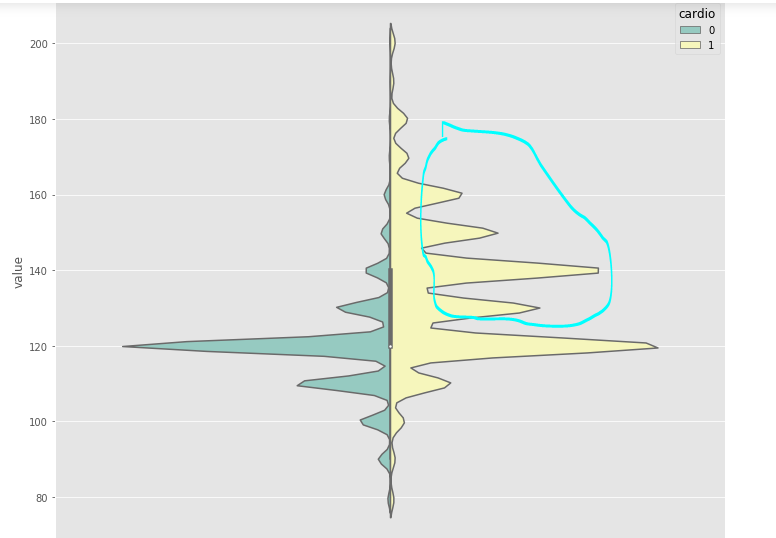Similar to Diastolic Blood Pressure, Systolic blood pressure is also linked to cardio disease.

### Bivariate: Height and Weight